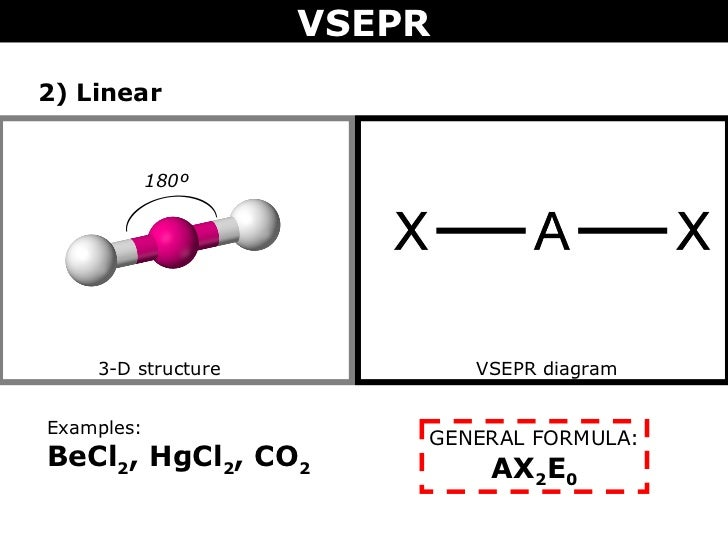# BeCl_2 and TeCl_2 are both covalent molecules, yet BeCl_2 is linear while TeCl_2 is bent. Why?

Dec 23, 2015

${\text{TeCl}}_{2}$: The central $\text{Te}$ atom has $6$ valence electrons. When the two $\text{Cl}$ atoms bond to the central atom, they each contribute one electron to form two sigma bonds. This leaves $4$ electrons on the central atoms in the form of $2$ lone electron pairs.

This is the VSEPR shape ${\text{AX"_2"E}}_{2}$, a tetrahedron shape with two electron pairs and two atoms, creating a bent molecular shape.${\text{BeCl}}_{2}$, on the other hand, has $\text{Be}$ as its central atom. Since $\text{Be}$ only has $2$ valence electrons, the two sigma bonds formed by the $\text{Cl}$ atoms leave $\text{Be}$ with no lone pairs.

The VSEPR shape is ${\text{AX"_2"E}}_{0}$ and forms a linear shape.